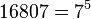# Groups of order 16807

## Contents

See pages on algebraic structures of order 16807| See pages on groups of a particular order

## Statistics at a glance

To understand these in a broader context, see: groups of order 7^n|groups of prime-fifth order

Since$16807 = 7^5$ is a prime power, and prime power order implies nilpotent, all groups of this order are nilpotent groups.

Quantity Value Explanation
Total number of groups 83 PORC function for number of groups of order$p^5$ for$p \ge 5$ is:$2p + 61 + 2\operatorname{gcd}(p-1,3) + \operatorname{gcd}(p-1,4)$. Plugging in$p = 7$ gives 83.
Number of abelian groups 7 equals the number of unordered integer partitions of$5$ (this is the$5$ appearing in the exponent part of$7^5$). See classification of finite abelian groups and structure theorem for finitely generated abelian groups.
Number of groups of nilpotency class exactly two 32 the general formula for order$p^5$ with$p \ge 5$ is$p + 25$.
Number of groups of nilpotency class exactly three 33 the general formula for order$p^5$ with$p \ge 5$ is$p + 26$.
Number of groups of nilpotency class exactly four, i.e., maximal class groups 11 the general formula for order$p^5$ with$p \ge 5$ is$3 + 2\operatorname{gcd}(p-1,3) + \operatorname{gcd}(p-1,4)$.

## GAP implementation

The order 16807 is part of GAP's SmallGroup library. Hence, any group of order 16807 can be constructed using the SmallGroup function by specifying its group ID. Unfortunately, IdGroup is not available for this order, i.e., given a group of this order, it is not possible to directly query GAP to find its GAP ID.

Further, the collection of all groups of order 16807 can be accessed as a list using GAP's AllSmallGroups function.

Here is GAP's summary information about how it stores groups of this order, accessed using GAP's SmallGroupsInformation function:

```gap> SmallGroupsInformation(16807);

There are 83 groups of order 16807.
They are sorted by their ranks.
1 is cyclic.
2 - 42 have rank 2.
43 - 76 have rank 3.
77 - 82 have rank 4.
83 is elementary abelian.

This size belongs to layer 9 of the SmallGroups library.
IdSmallGroup is not available for this size.```